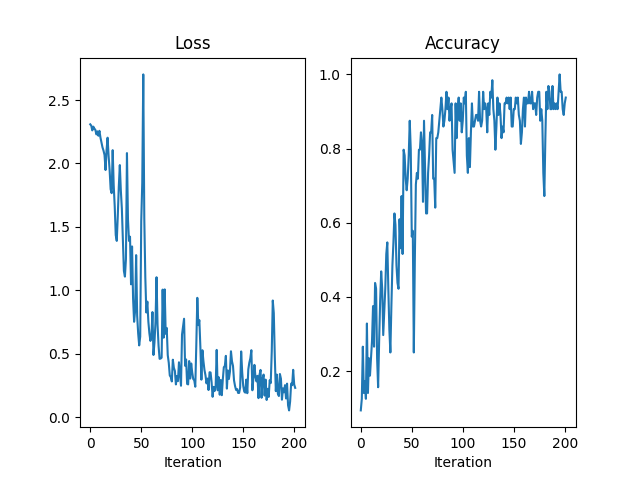# Differentially Private SGD.

Differentially Private Stochastic Gradient Descent (DP-SGD) [Abadi et al., 2016] is a rather simple idea. Instead of doing a typical SGD update, with the sum of all gradients in a minibatch,

$x' = x - \gamma g, \quad\quad g = \sum_i \underbrace{g_i}_{\text{individual gradients}},$

DP-SGD first truncates each individual gradient if their norm exceeds some threshold $$C$$ to ensure no single example influence the overall update too much,

$\tilde{g}_i = g_i / \max(1, \Vert g_i\Vert_2/C), \quad\quad \tilde{g} = \sum_i \tilde{g}_i,$

and adds Gaussian noise to the update,

$x' = x - \gamma (\tilde{g} + \epsilon), \quad\quad \epsilon \sim \mathcal{N}(0, C \sigma^2 I)$

That’s the TL:DR, anyway. It is not too difficult to get to an implementation of DP-SGD that works, but getting individual gradients from a minibatch in a way that scales can be tricky. This examples shows how to use the BatchGrad extension, which gives access to individual gradients.

Let’s get the imports, configuration and some helper functions out of the way first.

import matplotlib.pyplot as plt
import torch
import torch.nn as nn
from torch.optim import Optimizer

from backpack import backpack, extend

NUM_EPOCHS = 1
PRINT_EVERY = 50
MAX_ITER = 200
BATCH_SIZE = 64
DEVICE = torch.device("cuda:0" if torch.cuda.is_available() else "cpu")
torch.manual_seed(0)

"""Returns a view of v that can be broadcast with X.

If v is a one-dimensional tensor [N] and X is a tensor of shape
[N, ..., ], returns a view of v with singleton dimensions appended.

Example:
v is a tensor of shape  and X is a tensor of shape [10, 3, 3].
We want to multiply each [3, 3] element of X by the corresponding
element of v to get a matrix Y of shape [10, 3, 3] such that
Y[i, a, b] = v[i] * X[i, a, b].

w = make_broadcastable(v, X) gives a w of shape [10, 1, 1],
and we can now broadcast Y = w * X.
"""
broadcasting_shape = (-1, *[1 for _ in X.shape[1:]])

def accuracy(output, targets):
predictions = output.argmax(dim=1, keepdim=True).view_as(targets)
return predictions.eq(targets).float().mean().item()


We will use a small CNN with 2 convolutions, 2 linear layers, and feed it some MNIST data.

def make_small_cnn(outputs=10, channels=(16, 32), fc_dim=32, kernels=(8, 4)):
return nn.Sequential(
nn.ReLU(),
nn.MaxPool2d(2, stride=1),
nn.ReLU(),
nn.MaxPool2d(2, stride=1),
nn.Flatten(),
nn.Linear(channels * 4 * 4, fc_dim),
nn.ReLU(),
nn.Linear(fc_dim, outputs),
)

model = make_small_cnn().to(DEVICE)
loss_function = nn.CrossEntropyLoss().to(DEVICE)


and we need to extend the model so that BackPACK knows about it.

model = extend(model)


Before writing the optimizer class, let’s see how we can use BackPACK on a single batch to compute the clipped gradients, without the overhead of the optimizer class.

We take a single batch from the data loader, compute the loss, and use the with(backpack(...)) syntax to activate two extensions; BatchGrad and BatchL2Grad.

x, y = next(iter(mnist_dataloader))
x, y = x.to(DEVICE), y.to(DEVICE)

loss = loss_function(model(x), y)
loss.backward()


BatchGrad computes individual gradients and BatchL2Grad their norm (squared), which get stored in the grad_batch and batch_l2 attributes of the parameters

for p in model.parameters():
print(
"{:28} {:32} {}".format(
)
)

torch.Size([16, 1, 8, 8])    torch.Size([64, 16, 1, 8, 8])    torch.Size()
torch.Size()             torch.Size([64, 16])             torch.Size()
torch.Size([32, 16, 4, 4])   torch.Size([64, 32, 16, 4, 4])   torch.Size()
torch.Size()             torch.Size([64, 32])             torch.Size()
torch.Size([32, 512])        torch.Size([64, 32, 512])        torch.Size()
torch.Size()             torch.Size([64, 32])             torch.Size()
torch.Size([10, 32])         torch.Size([64, 10, 32])         torch.Size()
torch.Size()             torch.Size([64, 10])             torch.Size()


To compute the clipped gradients, we need to know the norms of the complete individual gradients, but ad the moment they are split across parameters, so let’s reduce over the parameters

l2_norms_squared_all_params = torch.stack([p.batch_l2 for p in model.parameters()])
l2_norms = torch.sqrt(torch.sum(l2_norms_squared_all_params, dim=0))


We can compute the clipping scaling factor for each gradient, given a maximum norm C,

$\begin{split}\\max(1, \Vert g_i \Vert/C),\end{split}$

as a tensor of [N] elements.

C = 0.1
scaling_factors = torch.clamp_max(l2_norms / C, 1.0)


All that remains is to multiply the individual gradients by those factors and sum them to get the update direction for that parameter.

for p in model.parameters():


## Writing the optimizer

Let’s do the same, but in an optimizer class.

class DP_SGD(Optimizer):
"""Differentially Private SGD.

Arguments:
params (iterable): iterable of parameters to optimize or dicts defining
parameter groups
lr (float, optional): coefficient that scale delta before it is applied
to the parameters (default: 1.0)
max_norm (float, optional): maximum norm of the individual gradient,
to which they will be clipped if exceeded (default: 0.01)
stddev (float, optional): standard deviation of the added noise
(default: 1.0)
"""

def __init__(self, params, lr=0.1, max_norm=0.01, stddev=2.0):
self.lr = lr
self.max_norm = max_norm
self.stddev = stddev
super().__init__(params, dict())

def step(self):
"""Performs a single optimization step.

The function expects the gradients to have been computed by BackPACK
and the parameters to have a batch_l2 and grad_batch attribute.
"""
l2_norms_all_params_list = []
for group in self.param_groups:
for p in group["params"]:
l2_norms_all_params_list.append(p.batch_l2)

l2_norms_all_params = torch.stack(l2_norms_all_params_list)
total_norms = torch.sqrt(torch.sum(l2_norms_all_params, dim=0))
scaling_factors = torch.clamp_max(total_norms / self.max_norm, 1.0)

for group in self.param_groups:
for p in group["params"]:
)

noise_magnitude = self.stddev * self.max_norm



## Running and plotting

We can now run our optimizer on MNIST.

optimizer = DP_SGD(model.parameters(), lr=0.1, max_norm=0.01, stddev=2.0)

losses = []
accuracies = []
for epoch in range(NUM_EPOCHS):
for batch_idx, (x, y) in enumerate(mnist_dataloader):
x, y = x.to(DEVICE), y.to(DEVICE)

outputs = model(x)
loss = loss_function(outputs, y)

loss.backward()

optimizer.step()

# Logging
losses.append(loss.detach().item())
accuracies.append(accuracy(outputs, y))

if (batch_idx % PRINT_EVERY) == 0:
print(
"Epoch %3.d/%d Iteration %3.d " % (epoch, NUM_EPOCHS, batch_idx)
+ "Minibatch Loss %.3f  " % losses[-1]
+ "Accuracy %.3f" % accuracies[-1]
)

if MAX_ITER is not None and batch_idx > MAX_ITER:
break

Epoch   0/1 Iteration   0 Minibatch Loss 2.309  Accuracy 0.094
Epoch   0/1 Iteration  50 Minibatch Loss 1.543  Accuracy 0.562
Epoch   0/1 Iteration 100 Minibatch Loss 0.343  Accuracy 0.875
Epoch   0/1 Iteration 150 Minibatch Loss 0.265  Accuracy 0.891
Epoch   0/1 Iteration 200 Minibatch Loss 0.255  Accuracy 0.906

fig = plt.figure()

axes.plot(losses)
axes.set_title("Loss")
axes.set_xlabel("Iteration")

axes.plot(accuracies)
axes.set_title("Accuracy")
axes.set_xlabel("Iteration")Text(0.5, 23.52222222222222, 'Iteration')


Total running time of the script: ( 0 minutes 10.613 seconds)

Gallery generated by Sphinx-Gallery